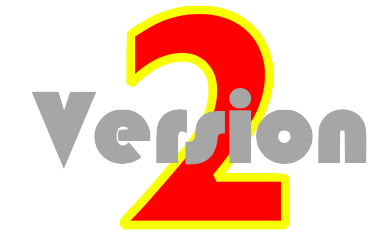### 1-Dimensional Kinematics

A Concept-Builder is an interactive questioning module that presents learners with carefully crafted questions that target various aspects of a concept. Each Concept Builder focuses the learner's attention upon a discrete learning outcome. Questions target that outcome from a variety of angles using multiple difficulty levels or varying activities. Learners ponder the given information and answer questions in the hopes of earning Stars and Medals. These Stars (for answering questions) and Medals (for completing levels or activities) are displayed on the screen, allowing a teacher to track student progress. The following Concept Builders target concepts in 1-Dimensional Kinematics.

Distance vs. DisplacementLearning Goal:  To analyze a multi-stage motion and to determine the distance and the displacement for that motion.

AccelerationLearning Goal:  To identify whether an object accelerates or not, to determine the direction of the acceleration, and to determine the acceleration value if given simple numerical data.

Name That MotionLearning Goal:  To use an understanding of the language of kinematics in order to accurately match an animation of a motion to the corresponding verbal description of that motion.

Motion DiagramsLearning Goal:  To construct the motion diagram for a given motion, showing properly-spaced dots, velocity vectors, and acceleration vectors.

Graph That MotionLearning Goal:  To use an understanding of the principles of kinematic graphing in order to accurately match an animation of a motion to the corresponding position-time graph or velocity-time graph of that motion.

Match That GraphLearning Goal:  To analyze the position-time graph for an object's motion and to match it to the corresponding velocity-time graph ... or vice versa.

Position-Time Graphs - Conceptual AnalysisLearning Goal:  To analyze a position-time graph and associate the graph features with motion characteristics of a moving object.

Position-Time Graphs - Numerical AnalysisLearning Goal:  To analyze a position-time graph in order to determine the object’s disance traveled, displacement, and velocity.

Velocity-Time GraphsLearning Goal:  To relate a verbal description of an object’s motion to the corresponding velocity-time graph representing the motion.

Dots and GraphsLearning Goal:  To analyze a dot diagram and match the motion that it describes to the appropriate position-time or velocity-time graph.​

Words and GraphsLearning Goal:  To identify the velocity-time graph that matches the verbal description given for an object’s motion.

Which One Doesn't Belong?Learning Goal:  To interpret various representations of an object’s motion in order to identify which representation is not like the others.

Free FallLearning Goal:  To express an understanding of the magnitude and direction of the velocity and acceleration vector for a free-falling object over the course of its trajectory.

Up and DownLearning Goal:  To understand the conceptual and numerical nature of the velocity and acceleration vectors of an object that is launched vertically upward from the ground and travels through its full trajectory.